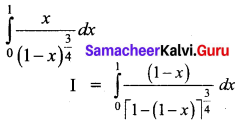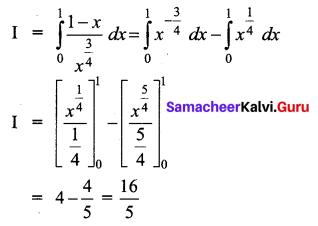# Samacheer Kalvi 12th Business Maths Solutions Chapter 2 Integral Calculus I Ex 2.9

Students can download 12th Business Maths Chapter 2 Integral Calculus I Ex 2.9 Questions and Answers, Samacheer Kalvi 12th Business Maths Book Solutions Guide Pdf helps you to revise the complete Tamilnadu State Board New Syllabus and score more marks in your examinations.

## Tamilnadu Samacheer Kalvi 12th Business Maths Solutions Chapter 2 Integral Calculus I Ex 2.9

Evaluate the following using properties of definite integrals:

Question 1.
$$\int_{-\frac{\pi}{4}}^{\frac{\pi}{4}} x^{3} \cos ^{3} x d x$$
Solution: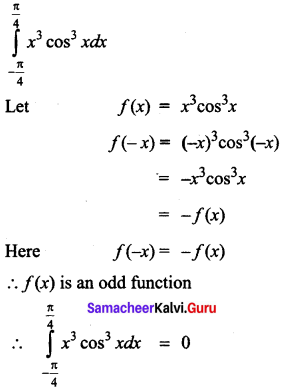Question 2.
$$\int_{-\frac{\pi}{2}}^{\frac{\pi}{2}} \sin ^{2} \theta d \theta$$
Solution: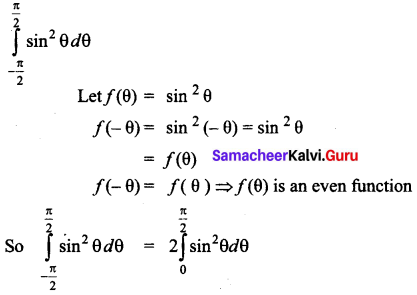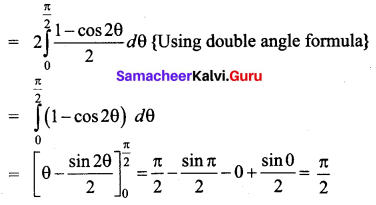Question 3.
$$\int_{-1}^{1} \log \left(\frac{2-x}{2+x}\right) d x$$
Solution: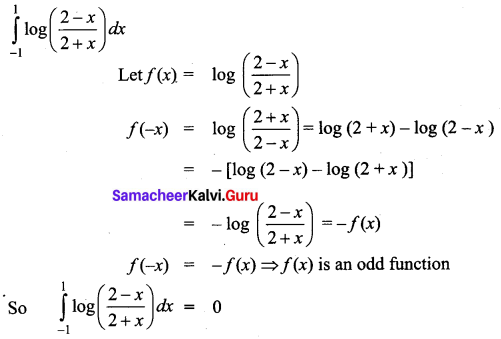Question 4.
$$\int_{0}^{\frac{\pi}{2}} \frac{\sin ^{7} x}{\sin ^{7} x+\cos ^{7} x} d x$$
Solution: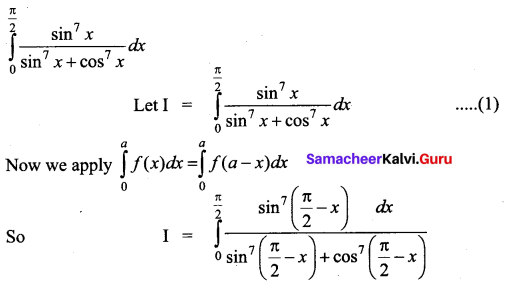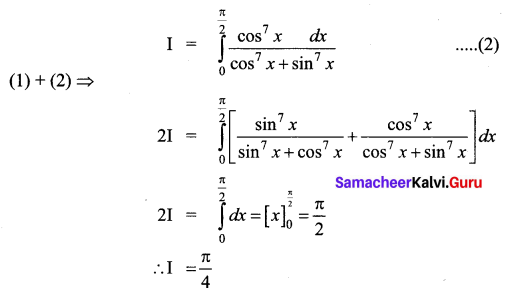Question 5.
$$\int_{0}^{1} \log \left(\frac{1}{x}-1\right) d x$$
Solution: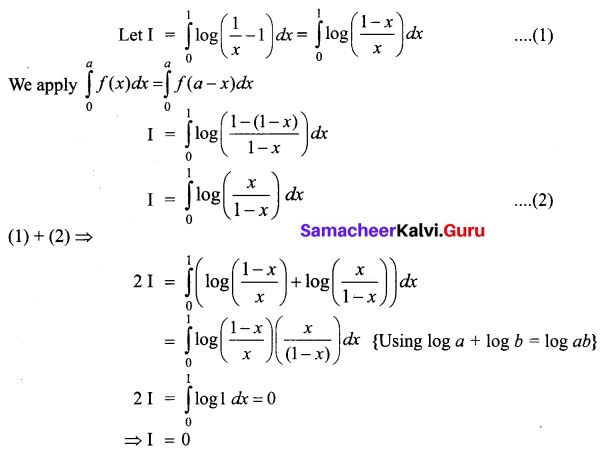Question 6.
$$\int_{0}^{1} \frac{x}{(1-x)^{\frac{3}{4}}} d x$$
Solution: